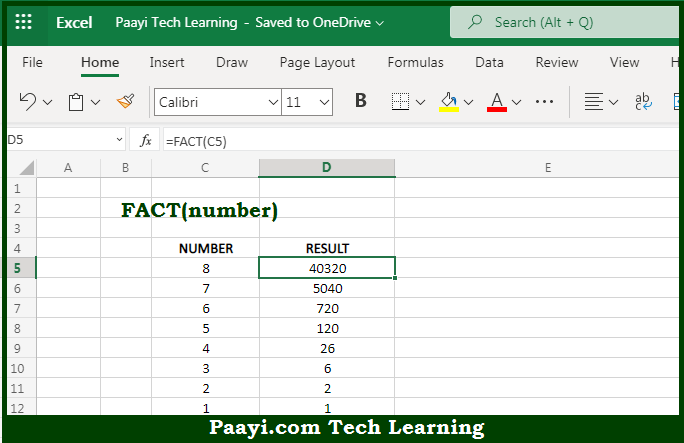# Learn How to Use Microsoft Excel FACT Function

Written by | 0 Comments | 626 Views

In this article, you will learn how to use the Microsoft Excel FACT function and its prime function in Microsoft Excel. You will also get to know the Microsoft Excel FACT function return value and syntax with the help of some examples.

Microsoft Excel FACT Function

The main function of the Microsoft Excel FACT function is used to find the factorial of a number. So, with the help of the FACT function, you can able to return the factorial of the given number. Let's say for example - =FACT(4), will return 24, as 4x3x2x1. So, with the help of the Microsoft Excel FACT function, you can easily find the factorial of the number.

Return Value of FACT Function

The return value will be the factorial of a number.

Syntax of FACT Function

=FACT(number)

Where the arguments:

• number: This is the number of which you want to find out the factorial.

How to Use Microsoft Excel FACT Function?So we know that the Microsoft Excel FACT function is used to you can able to return the factorial of the given number. Let's say for example - =FACT(4), will return 24, as 4x3x2x1. It should be noted that the number cannot be negative otherwise the FACT function will return the #NUM error. In the same way, if the number is not an integer it will be truncated to an integer, then solved. So, with the help of the Microsoft Excel FACT function, you can easily find the factorial of the number.